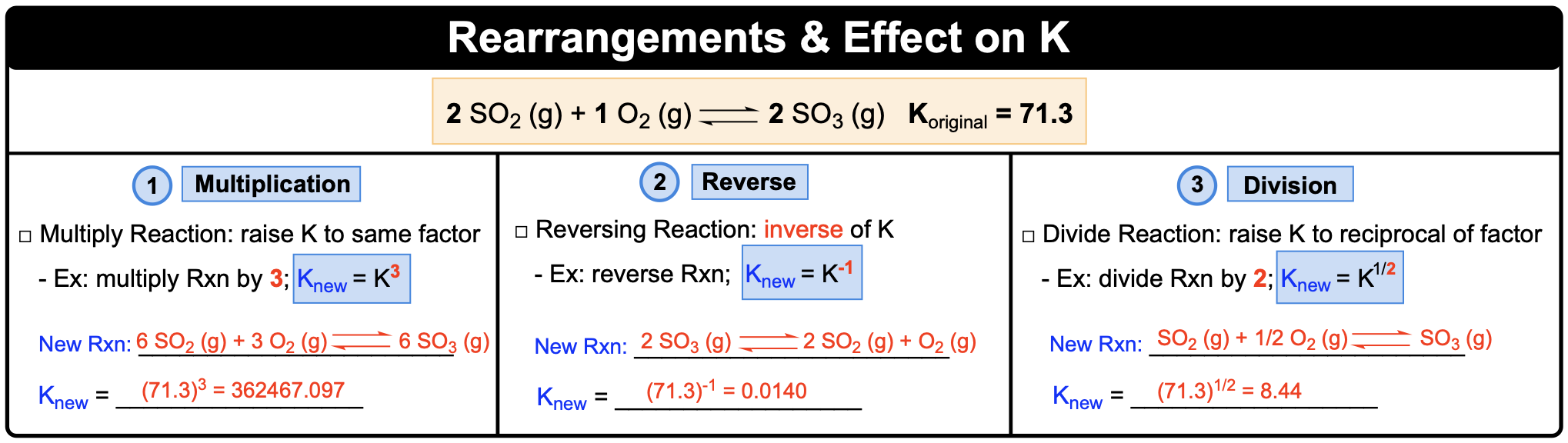Clutch Prep is now a part of Pearson
Ch.14 - Chemical EquilibriumWorksheetSee all chapters

# Using Hess's Law To Determine K

See all sections
Sections
Intro to Chemical Equilibrium
Equilibrium Constant (K)
Equilibrium Constant Calculations
Kp and Kc
Using Hess's Law To Determine K
Calculating K For Overall Reaction
Le Chatelier's Principle
ICE Charts
Reaction Quotient

Using Hess's Law concept to determine K of a reaction.

###### Equilibrium Constant and Hess's Law

Concept #1: Reaction Rearrangements and Effect on value of KExample #1: Given the reaction: 2 Cl2 (g) + 2 H2O (g) ⇌ 4 HCl (g) + O2 (g) Kp = 7.5x10-2 , calculate Kp of the reactions below.

a) Cl2 (g) + H2O (g) ⇌ 2 HCl (g) + ½ O2 (g)  _____________

b) 4 HCl (g) + O2 (g) ⇌ 2 Cl2 (g) + 2 H2O (g)  _____________

c) 16 HCl (g) + 4 O2 (g) ⇌ 8 Cl2 (g) + 8 H2O (g)  _____________

Practice: Kc = 6.5 x 102 at a particular temperature for a reaction: 2 NO(g) + 2 H(g) ⇌ N2(g) + 2 H2O(g). Calculate Kc at same temperature for the following reaction: 1/3 N2(g) + 2/3 H2O(g) ⇌ 2/3 NO(g) + 2/3 H(g).